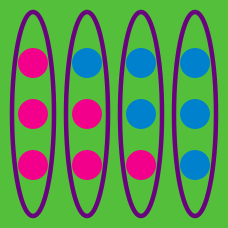Probability

# Combinations with Repetition

A bakery sells three kinds of pastries: donuts, muffins, and cookies. If you go to the store to buy $28$ pastries, how many different combinations of them can you buy if they only have $27$ of each type available?

Details and assumptions

All the pastries of one type are indistinguishable.

For his reverse birthday party, George is giving away bags with toys in them. If the party store sells 4 different toys and George is making $9$ bags, each with a single toy in it, how many different combinations of toys can George make?

How many ways are there to choose distinct numbers $a, b$ and $c$ where $a < b < c$ from among the integers $\{1, 2, \ldots, 110\}$ such that $a+b+c$ is an even number?

Cards for a trading card game can be purchased in packages of 6 cards, of which exactly 1 of them is a rare card.

If there are a total of 8 rare cards that can be collected, and you purchase $3$ packages of cards, how many different combinations of the rare cards could you end up with?

For example, you could have 1 of the second rare cards, and the rest are the eighth rare card.

You have 6 identical balls and 6 (distinct) boxes numbered $1$ through $6.$ How many ways can the 6 balls be distributed amongst the boxes?

Details and assumptions

Some of the boxes can be empty.

×